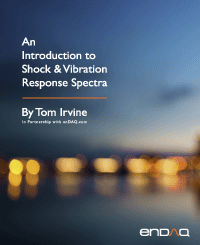Fourier Transforms
By Tom Irvine
SECTION 1

# Fourier Transform Equations

SECTION 2

## Textbook Fourier Transform

The Fourier transform is a method for identifying the spectral content of a time history. This transform has already been used previously in this document, including for the channel beam vibration response in Figure 11.3 and Figure 11.4. This section will give background equations starting with the rarefied mathematician approach and finishing with the practical engineering version.

The textbook Fourier transformfor a continuous time series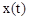is defined as

Note that. And both the time and frequency domains are considered to extend from minus to plus infinity in their respective spheres.

There is also an inverse Fourier transform defined as

As an aside, the corresponding Euler’s equation is

There are several impractical quirks begat from this transform and inverse pair. One is that a signal as simple as a pure sine function will have a spectral component at a negative of its true frequency, as shown in Figure 15.1. How is an engineer to design and test for a negative frequency? In addition, measured time histories have a distinct start and stop time with a finite duration. Next, integration is analogous to multiplication. Assume that x(t) represents an acceleration time history. The resulting Fourier transform from equation (1.1) would return a transform with amplitude dimension [acceleration-time]. What would this be? Velocity?

The last peculiarity is that measured time histories are strictly real. The resulting Fourier transform of a measured signal from equation (1.1) would be complex, with real and imaginary components. Now take this Fourier transform and insert it into the inverse formula in (1.2). The resulting time history will be complex. Fortunately, this concern is easily resolved because the imaginary components will all be zero.

The time history behind the imagery Fourier transform equation (1.1) is

The real Fourier transform is omitted because it is zero for a pure sine function with zero phase angle. The symbol δ represents the Dirac delta function, a mathematical oddity unsuitable for engineering design and test purposes.

Note the following traits for any given signal:

• The real Fourier transform is symmetric about the f=0 line.
• The imaginary Fourier transform is anti-symmetric about the f=0 line.
Discrete Fourier Transform

The discrete Fourier transform rectifies some, but not all, impracticalities of the textbook Fourier transform. Engineers collect data using data acquisition systems which return digitized outputs for measured analog inputs. The integral in equation (1.1) thus needs to be exchanged for a series, which is easily done. The resulting discrete Fourier transform is

Its corresponding inverse is

Eureka! Equation (1.5) does away with negative frequencies. The starting frequency will now be zero. Also, the Fourier transform amplitude dimension will now be the same that of the time history. The time domain dimension of acceleration will thus carry directly over to the Fourier transform. And both the time and frequency domain functions may now be defined over finite domains. Alas, this discrete pair will need further tweaking before the equations are ready for practical use, but progress has been made.

Recall the beloved sine function from equation (1.4). Set its amplitude at 1 G and its frequency at 1 Hz. Digitize it with a sample rate of 32 samples per second with a 512 second duration. The frequency step in the Fourier transform will be (1/512) Hz. The imaginary discrete Fourier transform is shown in the following figure.

The sine function has a frequency of 1 Hz. Again, the Fourier transform of a pure sine function is imaginary and antisymmetric. The antisymmetric reflection occurs about 16 Hz which is the Nyquist frequency, one-half the sample rate. Does the discrete Fourier transform imply that that a hypothetical component subjected to this environment needs to be designed and tested for 31 Hz as well as 1 Hz? How would the peak at 31 Hz change if the sample rate were increased to 64 samples per second? Is this some manifestation of Heisenberg uncertainty principle? And why is the absolute amplitude at 1 Hz equal to 0.5 but the sine function amplitude is 1?

The discrete Fourier transform in equation (1.5) will not be abandoned. It reappears in certain calculations such as going back-and-forth between impulse response functions and transfer functions, convolution applications, inverse Fourier transforms, etc. These intermediate concepts will be covered in a future document. But a more practical transform is needed presently.

Useful Discrete Fourier Transform

Sidestepping the quandaries raised in the previous section, let us modify the discrete Fourier transform into a practical tool. Henceforth, the Fourier transform will only be shown up to the Nyquist frequency. And the Fourier transform will be represented in terms of magnitude rather than real and imaginary components. The phase may also be shown with magnitude if needed. And the amplitudes at all nonzero frequencies will be doubled. A more elegant of way of expressing this is that the energy components above the Nyquist frequency will be folded about the Nyquist frequency to lower frequencies. The energy at 31 Hz in 15.2 would thus be deposited at 1 Hz. Note that the spectral magnitude at zero frequency is a special case since it remains the simple mean value of the signal.

The full amplitude Fourier transform up to the Nyquist frequency is

The beloved sine function’s Fourier magnitude is plotted in the above figure using equation (1.6). A clean spectral peak of 1 G appears at 1 Hz. The phase angle is omitted because it would only be valid at the sinusoid’s own frequency. It would be noise at other frequencies. There is a need for phase angle in other situations, such as modal testing, comparing the lag from input to response, etc.

SECTION 3
Leakage & Hanning Window
Leakage Error

There are numerous error sources associated with the Fourier transform, including "leakage,” which is a smearing of energy throughout the frequency domain. Leakage results when both of the following conditions are present:

• The signal is taken over a finite duration
• The signal is "non-periodic" in the time record

Both these conditions are usually present in engineering data, so leakage is common. For example, leakage occurs if a Fourier transform is calculated for a non-integral number of sine function cycles.

Consider that a data acquisition system is used to monitor a sine function over a 10 second duration. The sine function has an amplitude of 1 G and a frequency of 1 Hz, as shown in the top subplot. The sample rate is 32 samples per second. Now assume that the data acquisition system has a limited memory buffer and measures only three cycles as shown in the middle subplot. Note that the time history amplitude is zero at the start and end of the record, with an integer number of cycles. In essence, the Fourier transform will correctly assume that the original signal is a series of three-cycle segments as shown in the bottom subplot, such that no leakage occurs as shown in Figure 1.6.

Repeat the previous example but with a tighter memory buffer that only measures 2 1/2 cycles as shown in the middle subplot. Leakage will occur due to the non-integer number of cycles. The Fourier transform will assume that the original signal is a distorted series of these segments as shown in the bottom subplot, as shown in Figure 1.6.

The top subplot shows a clean spectral line at 1 Hz for the case of three whole cycles. The bottom subplot shows that the energy is smeared across numerous bands as a result of leakage error from the non-integer number of cycles.

On a related note, the top subplot has a spectral line available at exactly 1 Hz. But the bottom subplot lacks a line at 1 Hz and instead has lines at 0.8 and 1.2 Hz. Recall that the frequency step is the inverse of the duration. The three-cycle spectral increment is thus 0.333 Hz, whereas the two and half cycle step is 0.4 Hz.

Hanning Window

The leakage error can be reduced by subjecting the time history to an appropriate window. Two common types of windows are the rectangular window and the Hanning window.

The rectangular, or flat, window leaves the time history data unmodified and is equivalent to no window at all. A rectangular window is appropriate for transient data or nonstationary data, including the type of shock time histories presented in Section 0. It does not reduce leakage.

One of the most common windows is the Hanning window, or the cosine squared window. It works best with stationary data. This window tapers the time history data so that the amplitude envelope decreases to zero at both the beginning and end of the time segment. The Hanning window w(t) can be defined the is plotted in Figure 1.7.

A normalization factor of the square root of (8/3) may be applied to the Hanned data to compensate for lost energy, with the goal of maintaining the original overall RMS level.

A sine function before and after Hanning window application is show in the above figure. The non-integer number of cycles will cause a leakage error which is mitigated by the Hanning window although not eliminated. The Fourier transforms of each are shown in Figure 1.9.Figure 3.6. Fourier Spectra, Sine Function Before and After Hanning Window

Ideally, the Fourier transform would have a single, discrete line at 1 Hz with an amplitude of 1 G. A challenge is that there are spectral lines available at 0.945 Hz and 1.05 Hz but none at 1.0 Hz. Both the rectangular and Hanning Fourier transforms have some leakage error as a result. The rectangular window produces more leakage error than the Hanning window. Thus, the Hanning window is recommended for stationary data.

SECTION 4
Fast Fourier Transform
FFT Characteristics
 2 256 32,768 4 512 65,536 8 1024 131,072 16 2048 262,144 32 4196 524,288 64 8192 1,048,576 128 16,384 2,097,152

The discrete Fourier transform requires a tremendous amount of calculations for digital signals with large numbers of points. A time history with M coordinates would require M2 complex multiplication steps. The discrete Fourier transform can be carried out by a Fast Fourier transform (FFT) method, however. The method is based on a time series with a number of points equal to 2N, where N is an integer. The FFT requires M log 2 M complex multiplication steps, where M = 2N . Some length examples are shown in Table 1.1.

FFT Example

Now consider a time history with 1,000,000 points. A regular Fourier transform would require 1012 complex multiplication steps. On the other hand, an FFT would only require approximately 2(107) steps. Thus, the FFT achieves the calculation in 1/50,000 th of the time.

The above example is not quite correct. Again, the FFT is based on a time series with 2N coordinates. Note that 219 = 524,288 and 220 = 1,048,576. But a time history with 1,000,000 points falls between these two cases.

There are some options for dealing with a time history that is not an integer power of 2. One option is to truncate the time history. This should be acceptable if the data is stationary. In the above example, the time history would thus be truncated to 524,288 points. The second option is to pad the time history with trailing zeroes to bring its length to an integer power of 2. A problem with this option is that it artificially reduces the amplitude of the Fourier transform spectral lines. A third option is to carefully perform linear interpolation on the data so that its length becomes 2N. Or the problem can be circumvented altogether by configuring the data acquisition system to collect exactly 2N points. This was a feature of some vintage systems.

FFT Algorithm Background

The Fourier transform requires multiplying the time points by complex weighting functions. These functions have a certain symmetry for the case of time series with 2N points. The calculation can thus be simplified by taking advantage of this symmetry using concepts such as the unit circle and the butterfly algorithm. A few introductory steps are given here. Define a weighting factor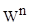.

The discrete Fourier transform becomes

The matrix representation is

Note that the weighting matrix in equation (1.11) is symmetric. Also note

The Fourier transform equation in matrix form simplifies to

The weights can be arranged geometrically as show in the following figure.Figure 4.1. Unit Circle for N=8, Polar Coordinates with Real and Imaginary Axes

The units circle shows some emerging symmetries. The real part of W1 is equal to that of W7. The imaginary part of W1 is equal to that of W3. Additional symmetries can be identified by noting that some values are the negative of others. Further development of the FFT algorithm is beyond the scope of this document but is given in References  and .

SECTION 5
Electrical Transformer Hum

Magnetic fields from alternating current induce vibration in certain materials, such as iron, steel, and ferrite alloys. This effect is called magnetostriction. Transformer hum is a common example of magnetostriction. This hum is a potential nuisance, and it is an energy loss mechanism.

A basic transformer has two sets of windings, the primary and the secondary. Certain transformers may have secondary windings or taps. The primary connects to the power source. The secondary connects to the load usually at a lower voltage. The windings are wrapped around a core, which may be iron or ferrite, depending on the design requirements. An iron core may be laminated with layers of iron and non-conducting material stacked together. A laminated iron core is used to reduce the creations of eddy currents in the iron core that would dissipate the energy being transferred from the primary coil to the secondary coil in the form of heat.

An alternating current is input to the primary winding. The current produces a magnetic flux, or magnetic lines of force in the core. The resulting magnetic field induces an alternating current in the secondary winding. The number of turns on each winding determines the output voltage from the transformer. The output voltage from the secondary is proportional to the ratio of the turns on the windings.

The magnetic field stresses the core material. The stress causes the core volume to expand regardless of the polarity. The core contracts to its equilibrium volume as the magnetic flux returns to zero. The material expands and contracts twice per each magnetic cycle, as shown in Figure 1.25. The core thus vibrates at 120 Hz in response to the changing magnetic field from 60 Hz AC power. The deformation amplitude may be so small that it is imperceptible to the unaided human eye. Nevertheless, it may be large enough to generate a hum. The hum frequency is 120 Hz, with integer harmonics. A hypothetical strain gage mounted on the core would output a rectified version of the curve in Figure 1.25.

The Fourier magnitude from a sound output of the transformer in Figure 1.11 is shown in Figure 1.14, with its 120 Hz peak and integer harmonics.

SECTION 6
Apache Helicopter Flyover Data

The Apache is a twin-engine army attack helicopter, as shown in Figure 1.15. It entered service with the US Army in 1984.

Helicopter noise consists of a complex mixture of sounds. The repeating impulse noise from the rotor blades is the dominant source of distinct spectral tones. The fundamental tone occurs at a characteristic frequency depending on the number of blades and their rotation speed. The blade-passing frequency (BPF) is the shaft rotation frequency times the number of rotor blades. Noise occurs at the BPF as well as at integer harmonics. Both the main rotor and the tail rotor generate this type of noise.

Rotor-vortex interaction noise is another source. This source is also referred to as blade-vortex interaction (BVI) noise. This source is significant in descent or level flight at low and medium velocities. Vortices form in the wake of the blades. A given rotor blade can run into the tip vortex shed by a preceding blade. This causes a distinctive type of noise: the annoying "blade-slap" of helicopters with slow-turning rotors, or a sharp fluttering noise for rotorcraft with fast-turning rotors.

Furthermore, the velocity at the tips of the advancing blades in high-speed forward flight may approach Mach 1. Shock waves begin to form at this condition, leading to shock induced flow separation. The shock waves and turbulent flow generate further noise. This source is referred to as highspeed impulsive (HSI) noise.

In addition, most helicopters are powered by turbine engines. Engine and gearbox noise consist of distinct spectral peaks as well as broadband random noise.

A segment of an acoustic time history from an Apache flyover is shown in the above figure. The signal is a summation of sine tones, mostly from the main and tail rotor blade passing frequencies.Figure 6.3. Apache Flyover Acoustic Fourier Spectra with Main Rotor BPFs Identified

The measured blade passing frequency is 21 Hz with integer harmonics. The Apache’s main rotor has four blades. The apparent main hub frequency is thus 5.25 Hz. The actual main hub frequency is @ 4.84 Hz per published data in Reference . The apparent frequency is higher due to a Doppler shift with the Apache flying toward the microphone.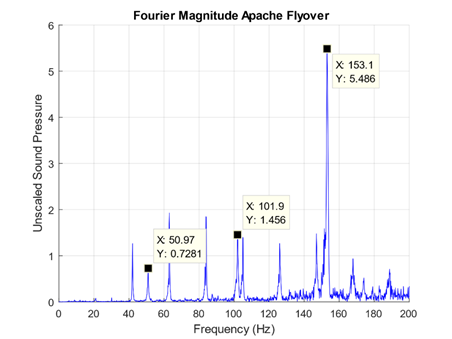Figure 6.4. Apache Flyover Acoustic Fourier Spectra with Tail Rotor BPFs Identified

The Apache tail rotor has four blades. The blades, however, are not oriented 90° (perpendicular) from each other as in most helicopters. Specifically, one set in front of the other at a 55° angle. The supplementary angle is 125°. This unusual arrangement is required because the two sets of blades use a "Delta-Hinge" which allows the blades to simultaneously flap and feather. The four blades appear to behave as two for the tail rotor blade pass frequency.

The purpose of this design is to render the blades more efficient and to reduce noise. The main rotor hub and the tail rotor hub are driven by the same source. Thus, there is a fixed ratio between the spin rates of each rotor. The tail rotor spin rate is about 4.86 times higher than the main rotor rate per Reference . Again, the measured, Doppler-shifted, main rotor spin rate is 5.25 Hz, or 315 rpm. The tail rotor spin rate is 25.5 Hz, or 1530 rpm, per the fixed ratio. The unique configuration of the blades yields a 2X blade passing frequency at 51 Hz. The blades behave as two pairs rather than as four individual blades. Tail rotor integer harmonics of this frequency occur at 102, 153, and 204 Hz. Recall that 153 Hz was the dominant peak in the Fourier transform in Figure 15.17, due to the tail rotor’s interaction with the main rotor wake.

SECTION 7
Turboprop Engine

The author once flew from Spokane to Seattle, Washington on an Alaska/Horizon Air Bombardier Q400 aircraft, similar to the one in Figure 15.19. He made an audio recording using his Android phone. The Fourier magnitude is shown in Figure 15.20. This model aircraft has two Pratt & Whitney Canada PW150A turboprop engines. The PW150A engine/propeller rotation rate during takeoff and climb is 1020 RPM but is throttled back at cruise altitude to 850 RPM, or 14.17 Hz. There are six blades on each engine, so the blade passing frequency is 85 Hz. This frequency and its integer harmonics are clearly visible in the spectral analysis.

SECTION 8
Waterfall FFT
Introduction

A waterfall FFT is a 3D plot with axes of time, frequency, and amplitude. It is useful for visualizing how spectral peak amplitudes and frequencies change with time. A spectrogram is a related 2D plot that shows this same data but with amplitude represented in terms of contour colors.

Solid Rocket Motor Example

Solid rocket motors may have standing pressure oscillations which form in the combustion chamber cavities, caused by vortex-shedding and other effects. This condition is called “Resonant Burn” or “Thrust Oscillation.” The sinusoidal oscillation frequency may sweep downward as the cavity volume increases due to the conversion of propellant to exhaust gas. A typical upper-stage motor susceptible to this problem in shown in Figure 1.21. Flight data from an accelerometer mounted on a bulkhead adjacent to this motor during its resonance burn is shown in Figure 1.22. The sinusoidal character is apparent in the close-up view.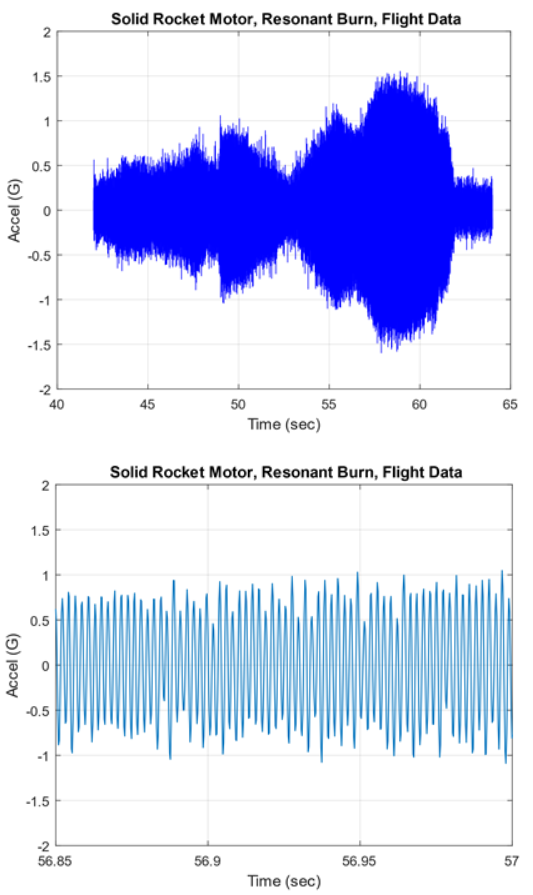Figure 8.2. Solid Motor Resonance Burn, Accelerometer Data, Full and Close-up Views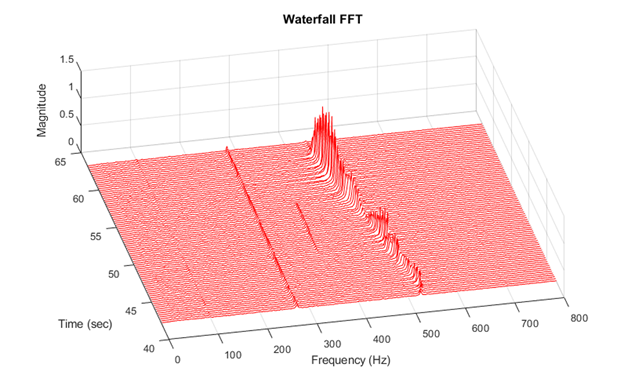Figure 8.3. Solid Motor Resonance Burn, Accelerometer Data, Waterfall FFT

The resonant burn spectral peaks begin at 520 Hz and then sweep downward to 450 Hz. The corresponding amplitude varies with both frequency and time probably due to structural resonance effects as measured by the bulkhead accelerometer. The causes of peaks at 280 and 350 Hz are unknown.

Wind Chime Acoustics

Recall the wind chime example from Section 8.1.7. The bending frequencies for the tallest chime were 238, 657, 1288 and 2127 Hz. A waterfall of the chime’s sounding is shown in Figure 1.24. The fundamental tone is very persistent with low damping. The second mode makes a very small contribution. The third mode initially has the highest generated sound, but the tone decays quickly. The fourth mode’s spectral peaks are barely visible in the plot.

SECTION 9
White Noise Fourier Transform

The Fourier transform is an excellent tool for resolving both the frequency and amplitude of a pure sinusoidal signal, aside from leakage concerns. Alas, it is a poor choice for random vibration. This is the motivation for representing random vibration in terms of power spectral density as presented in Section 16. The problem with the Fourier transform approach for random vibration relates to the dependence of the amplitude on the frequency resolution as shown in the following example. Consider a stationary white noise signal. An engineer may choose the entire signal or a representative segment for the Fourier transform calculation. A longer segment duration gives a finer frequency resolution. There would thus be more points in the Fourier transform magnitude to represent the signal’s energy. The corresponding amplitude of each point would be, on average, lower than if a shorter segment were used with a wider frequency resolution and fewer points.